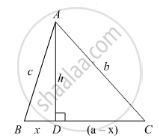Share

# In the Given Figure, ∠B < 90° and Segment Ad ⊥ Bc, Show that - Mathematics

Course

#### Question

In the given figure, ∠B < 90° and segment AD ⊥ BC, show that

(i) b= h+ a+ x- 2ax

(ii) b2 = a2 + c2 - 2ax#### Solution

(i) Since AD perpendicular to BC we obtained two right angled triangles, triangle ADB and triangle ADC.

We will use Pythagoras theorem in the right angled triangle ADC

AC2 = AD2 + DC2              ......(1)

Let us substitute AD = h, AC = b and DC = (a − x) in equation (1) we get,

b2 = h2 + (a − x)2

b2 = h2 + a2 − 2ax + x2

b2 = h2 + a2 + 𝑥2 − 2ax                ......(2)

(ii) Let us use Pythagoras theorem in the right angled triangle ADB as shown below,

AB2 = AD2 + BD2                       ......(3)

Let us substitute AB = c, AD = h and BD = x in equation (3) we get,

c2 = h2 + x2

Let us rewrite the equation (2) as below,

b2 = h2 + x2 + a2 - 2ax                ......(4)

Now we will substitute h2 + x2 = c2 in equation (4) we get,

b2 = c2 + a2 - 2ax

Therefore, b2 = c2 + a2 - 2ax.

Is there an error in this question or solution?

#### APPEARS IN

RD Sharma Solution for Class 10 Maths (2018 (Latest))
Chapter 7: Triangles
Ex. 7.7 | Q: 17 | Page no. 120
RD Sharma Solution for Class 10 Maths (2018 (Latest))
Chapter 7: Triangles
Ex. 7.7 | Q: 17 | Page no. 120

#### Video TutorialsVIEW ALL 

Solution In the Given Figure, ∠B < 90° and Segment Ad ⊥ Bc, Show that Concept: Application of Pythagoras Theorem in Acute Angle and Obtuse Angle.
S